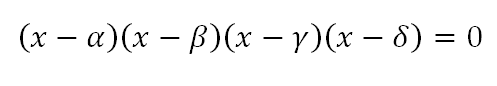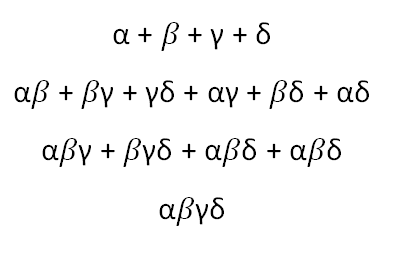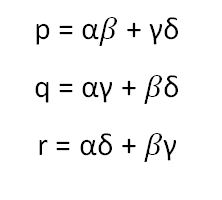## Tuesday, July 17, 2012

### Solving the Fourth Degree: Part 1

Today I'm going to try and write about the picturesque and mysterious way that the fourth degree equation is solvable. I've been using the Greek letters to stand for the roots of an equation, so the roots of the fourth degree will be alpha, beta, gamma, and delta. The factored equation can therefore be written explicitly asWhen you multiply this out, you get the coefficients of the polynomial as the elementary symmetric functions in alpha, beta, gamma, and delta:What makes the fourth degree equation solvable is the existence of this unique trio of functions in alpha, beta, gamma and delta:If we consider the splitting field of the polynomial, which is what I'm going to call the algebraic completion of the rationals formed by adjoining the four roots and taking all resulting algebraic combinations of those elements, it is clear that our p, q, and r reside within the splitting field. What is interesting is that they themselves are the roots of a cubic equation with rational coefficients! We can easily see why this must be so.

In the most general case, we cannot distinguish algebraically between the four roots of our quartic equation. If I state a true fact about alpha and beta, then you can turn around and state the same fact about gamma and delta, and as long as we restrict ourselves to algebraic manipulations whose final result is a rational number, then your fact will be just as true as my fact. In other words, we can freely shuffle our alpha, beta, gamma and delta and every algebraic statement is just as true before and after the shuffling.

Now, is we shuffle our four roots and consider the impact on our p's, q's, and r's, it is easy to verify the interesting result that any shuffling whatsoever will do one of two things: it will leave our p's and q's etc. untouched....or, it will swap them about with each other. In the even that there is swapping, it may be that just two of them get swapped, or it may be that all three are rotated with each other.

Furthermore, the property of indistinguishability with respect to shuffling of the four roots of the quartic is inherited by our p's and q's etc: a true statement about p, q, and r remains true if we arbitrarily shuffle them around. But this is just the property which characterises the roots of a cubic equation.

In fact, we can see explicitly that our p's and q's etc. are indeed the solution of a cubic equation by considering their elementary symmetric functions:

p + q + r

pq + qr + rp

pqr

These three functions are just the coefficients of the cubic equation of which p, q and r are the roots. We just need to substitute their definitions in terms of the alphas and betas etc. to verify that they are indeed rational numbers. This follows from the easily verified observation that they are symmetric in alpha, beta etc.

We've already seen that a cubic solution is solvable in radicals, and we talked about how to write the solution, That means we can get explicit expressions for p, q, and r.

We are now halfway to solving the quartic equation.